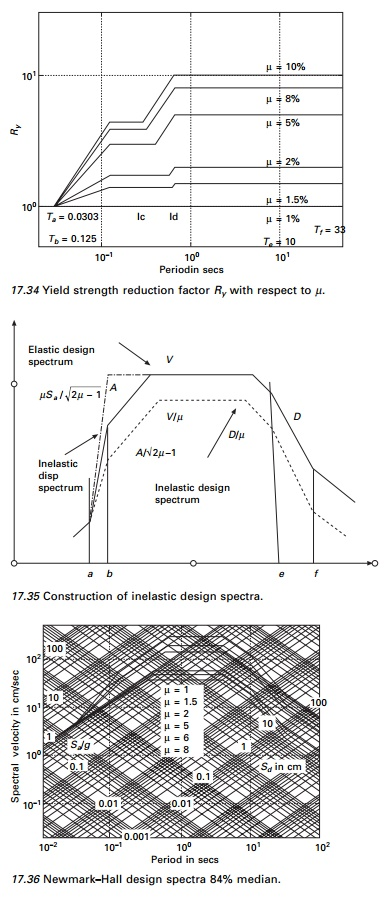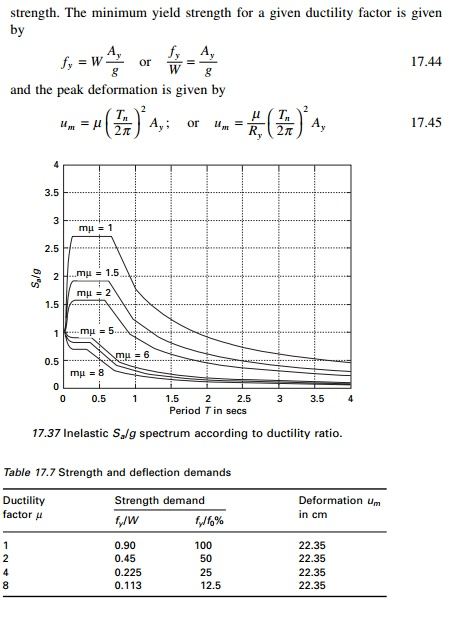Home | | Structural Dynamics and Earthquake Engineering | Application of inelastic design spectrum

# Application of inelastic design spectrum

The design deformation for an SDOF system can be determined for inelastic design spectrum given the parameters Tn, Žü and ┬Ą.

Application of inelastic design spectrum

The design deformation for an SDOF system can be determined for inelastic design spectrum given the parameters Tn, Žü and ┬Ą and design yieldwhere Ay is the pseudo-acceleration of the inelastic response spectrum. Consider an SDOF system with Tn = 1 s, Žü = 5% peak ground acceleration 0.5g. Table 17.7 gives strength and deflection demands.

There are two properties that must be considered while designing a structure, strength and ductility. One can design a very strong structure or a very ductile one or economic combination of both the properties. If the combination of strength and ductility is inadequate repairing such a structure is uneconomical or the structure will collapse.

Inelastic deformation

Assume we want to draw a curve relating Sa/g to D for a peak ground acceleration of 0.5g. The graph shown in Fig. 17.37 is converted to Ay versus D format, resulting in data pairs (Ay, D). Such a diagram is called demand diagram as shown in Fig. 17.38 for inelastic systems. Along the radial lines the period is constant. Superimposing on the demand curve, the load  deformation curve i.e. capacity curve for an elasto-plastic SDOF system, the ductility factor can be obtained from the intersection point of demand and capacity curves. This point provides the deformation demand.Study Material, Lecturing Notes, Assignment, Reference, Wiki description explanation, brief detail
Civil : Structural dynamics of earthquake engineering : Application of inelastic design spectrum |